# Fundamentals of Evolution

## Today's topics

1. The coalescent

2. Coalescent simulations

3. Structured coalescent

4. Species trees

4. Forward simulations

5. Assignment

### Wright-Fisher Model

Each generation randomly sample alleles from the parental generation. By incorporating a finite population size (N) into our sampling probabilities we can estimate the expected change in allele frequencies due to drift.

A discrete time model in which each generation is composed of 2N copies of each gene. Each subsequent generation 2N new copies are randomly drawn from the previous generation. The probability of obtaining k copies of allele p the next generation is:

$${{2N \choose k}p^{k}(1-p)^{2N-k}}$$

https://en.wikipedia.org/wiki/Genetic_drift#Wright.E2.80.93Fisher_model

### Wright-Fisher Model

A neutral evolutionary process (no selection) can be modeled using the WF model in which allele frequencies change over time by genetic drift.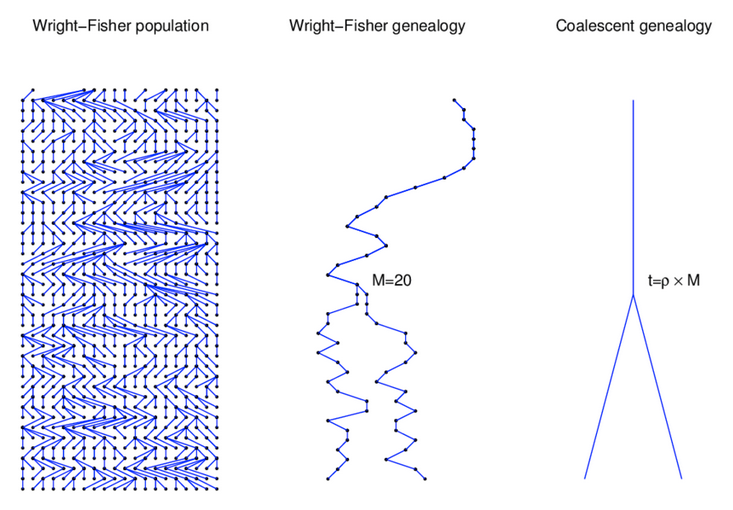Source: Alexei Drummond

### Characteristics of the coalescent

The coalescent is a mathematical description of the genealogical process arising in idealised populations.

It focuses on one or more genealogies (i.e., trees) underlying the history of a sample of chromosomes.

It is a probabilistic model, which implies that it describes the distribution of genealogies.

The principle idea is that genealogy holds all the information we need to know about our population (under a neutral evolutionary scenario).

### The coalescent for two sequences

In one generation these two sequences either came from the same parent ($\frac{1}{2N}$)
or they came from different parents ($1 - \frac{1}{2N}$)

The probability that these two sequences coalesced t generations ago can be calculated from these two probability statements:

$$\left(1 - \frac{1}{2N}\right)^{t - 1} \frac{1}{2N}$$

### The distribution of coalescent times

$$\mathrm{Pr}(\mathrm{coal}) = \binom{i}{2} \frac{1}{2N} = \frac{i(i-1)}{4N}$$
There are $\binom{i}{2}$ ways pairs of lineages can pick the same parent. Probability of coalescence scales quadratically with lineage count.

### Expected waiting time to coalescence

$$\mathrm{E}[T_i] = \frac{4N}{i(i-1)}$$
This is a geometric distribution. If each generation there is a $\frac{1}{x}$ probability of an event occurring, we expect to wait $x$ generations for the event to occur.

### Continuous time limit

With per-generation probability of an event $\frac{1}{x}$ small, but many generations, then the discrete time geometric distribution approximates to a continuous time exponential distribution.

Thus, we assume $T_i$ to be exponentially distributed with mean:

$$\mathrm{E}[T_i] = \frac{4N}{i(i-1)}$$

### Set of coalescent intervals with waiting times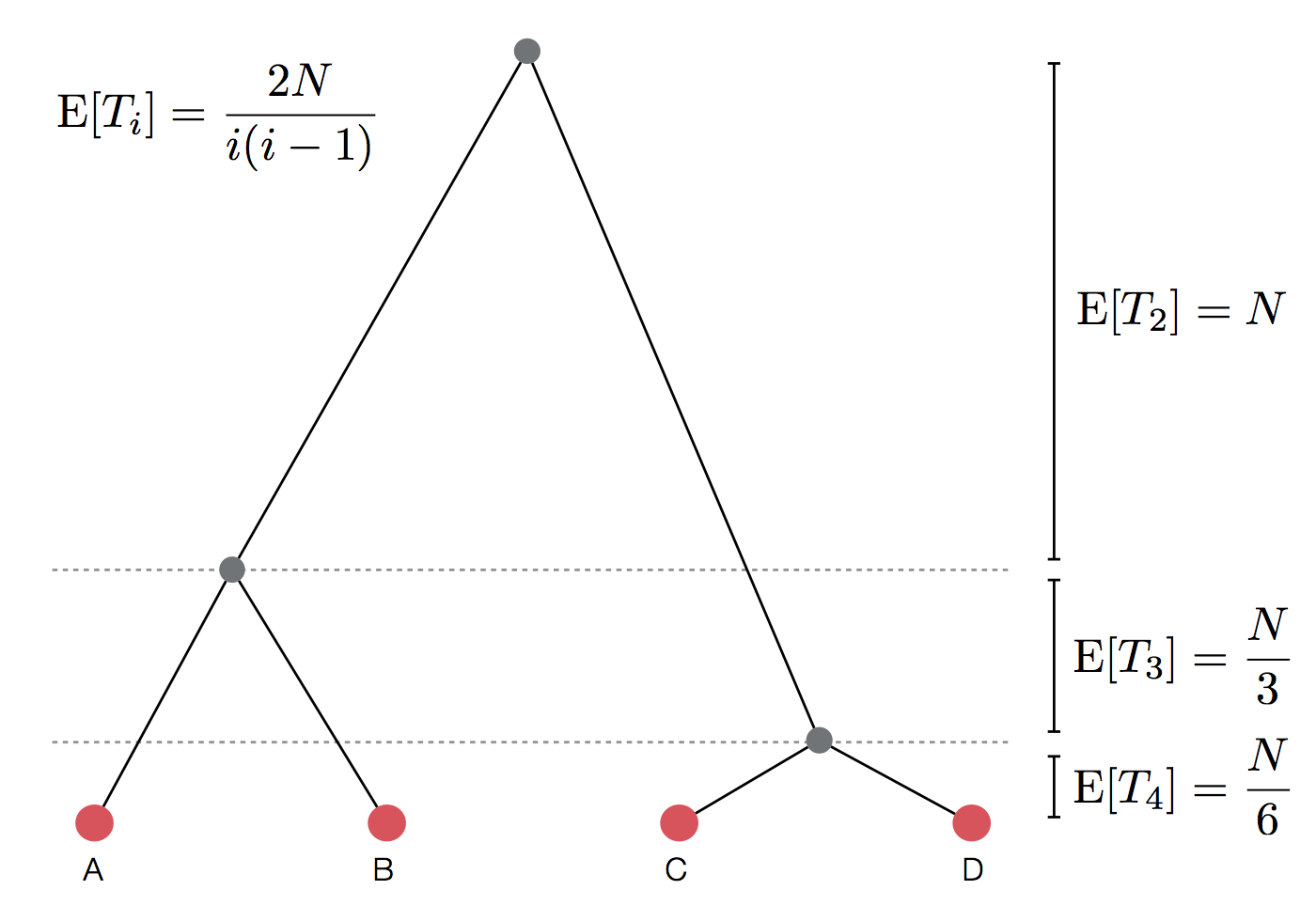### Set of coalescent intervals with waiting times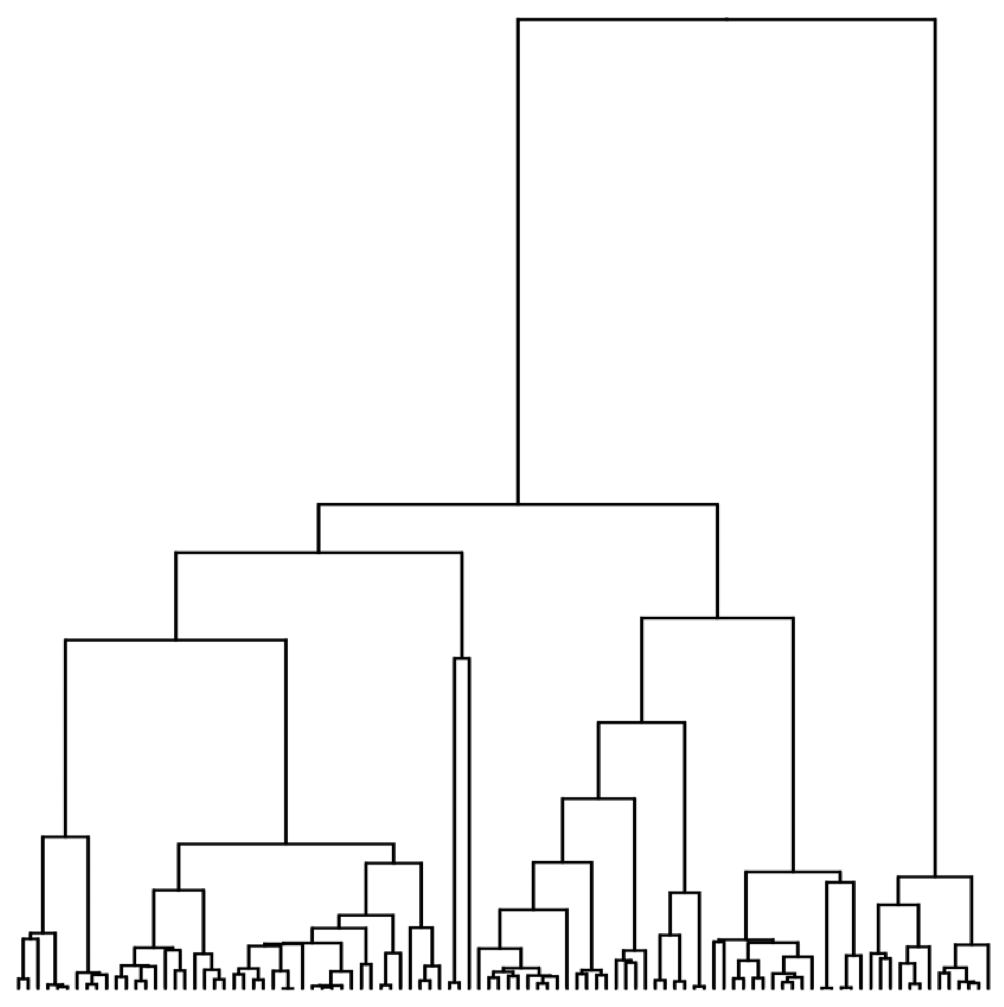### Set of coalescent intervals with waiting times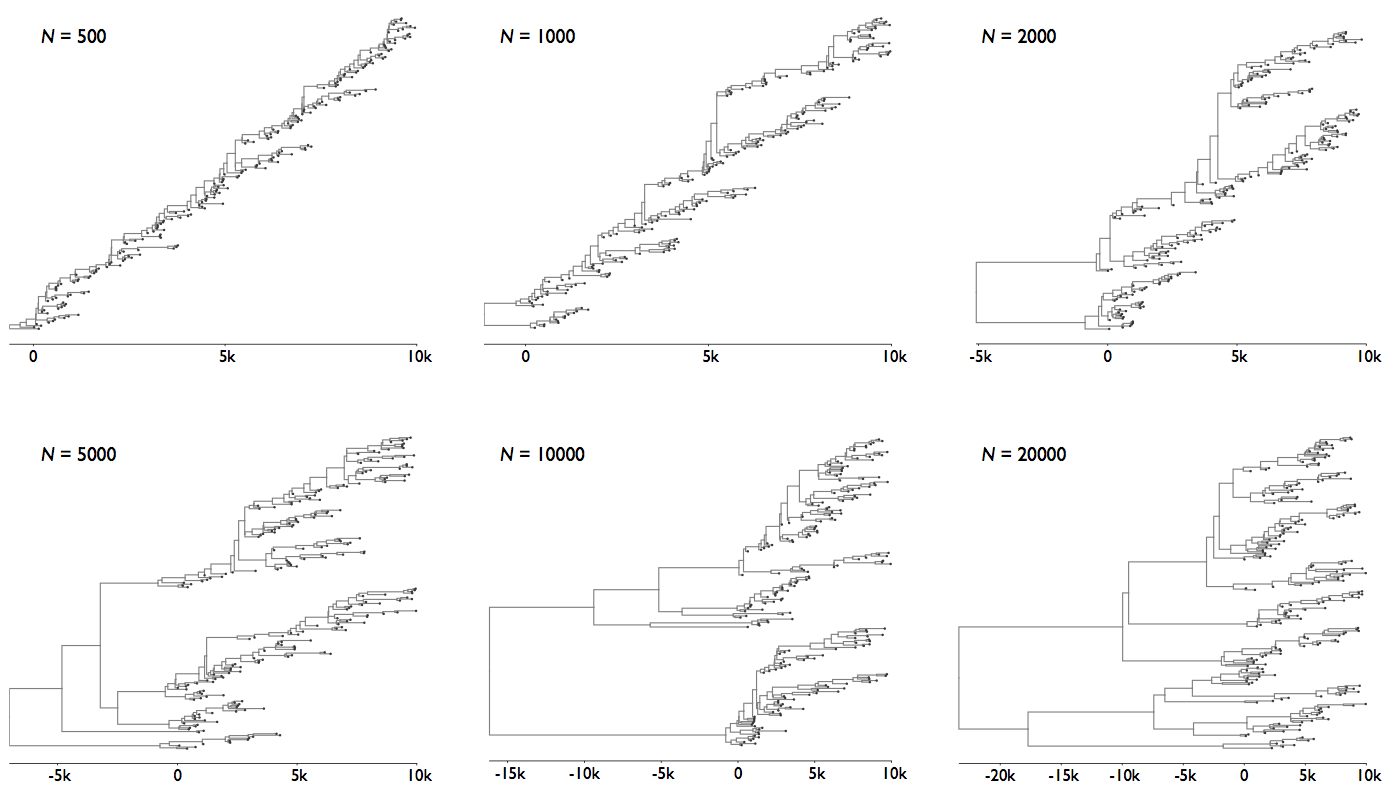### Pairwise genetic diversity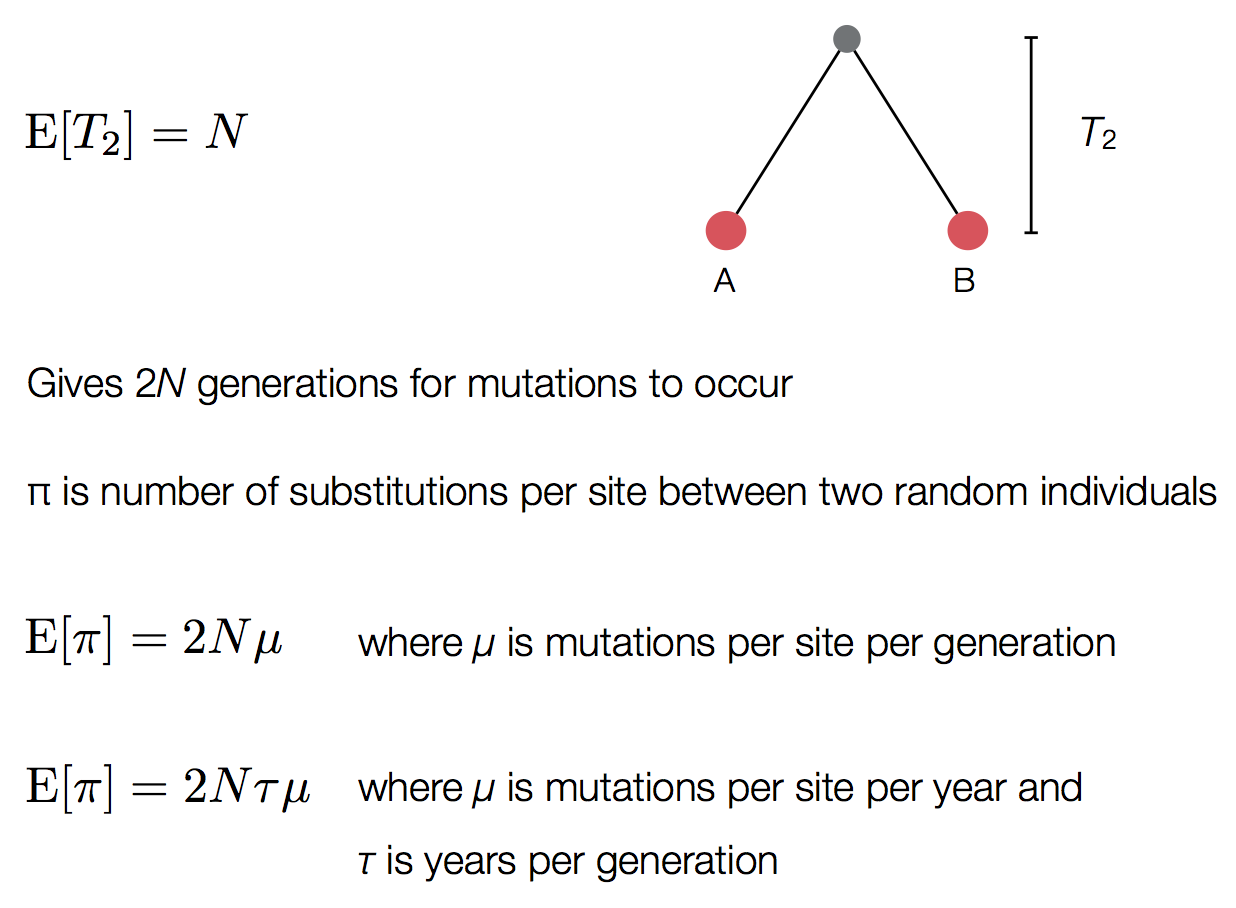### Time to the most recent common ancestor (TMRCA)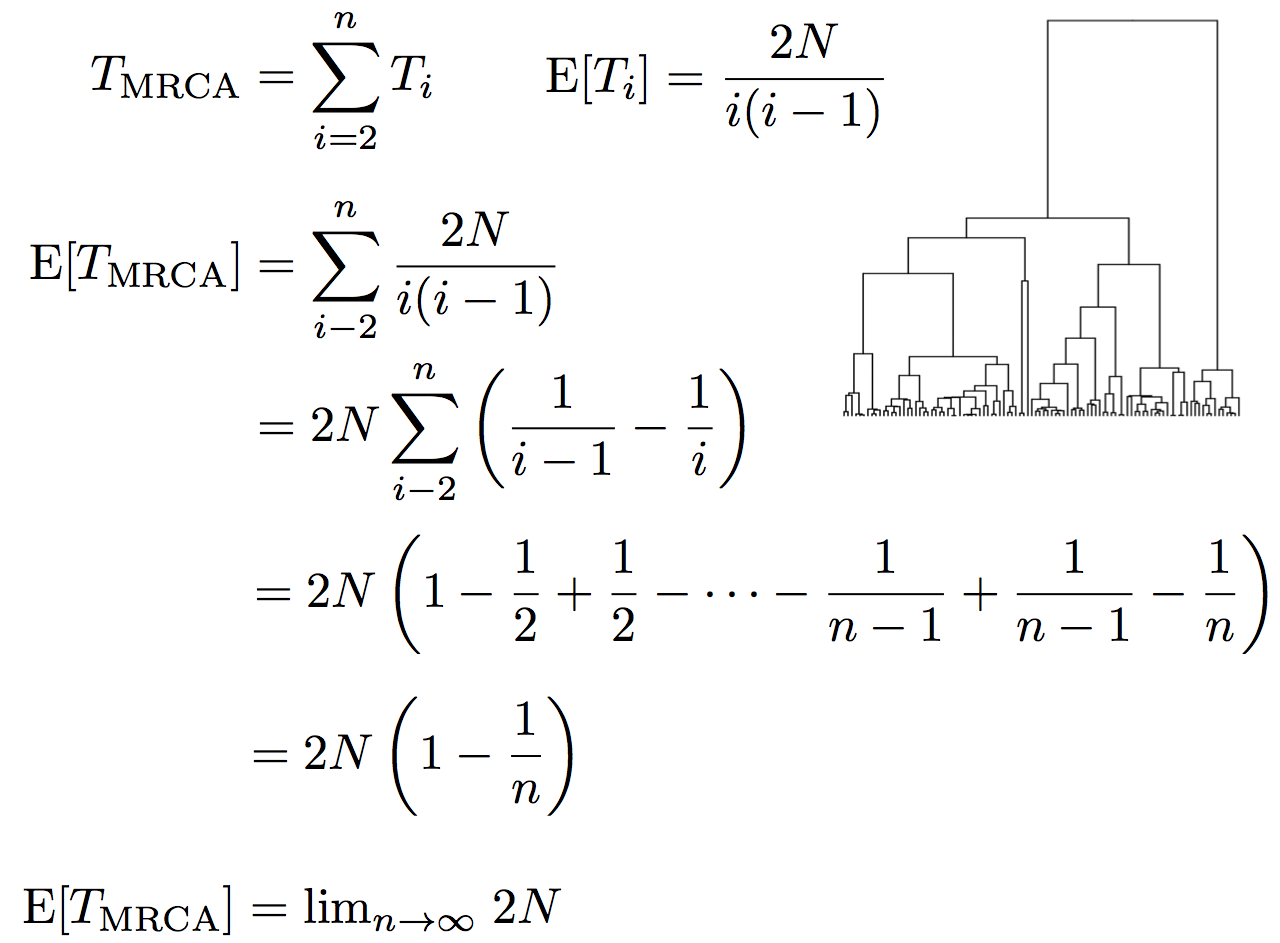### Coalescent patterns can estimate population growth/decline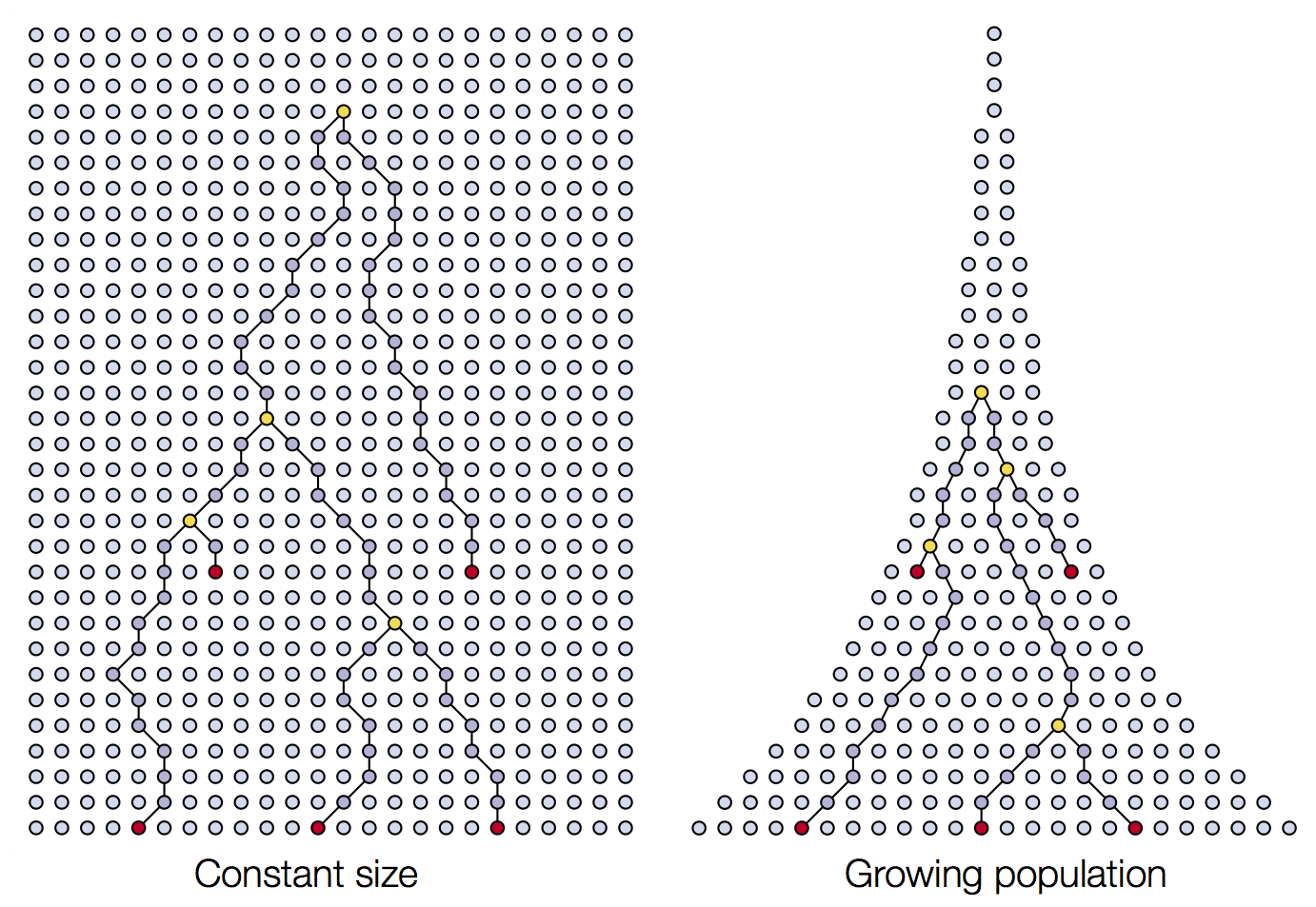### Coalescent patterns can estimate population growth/decline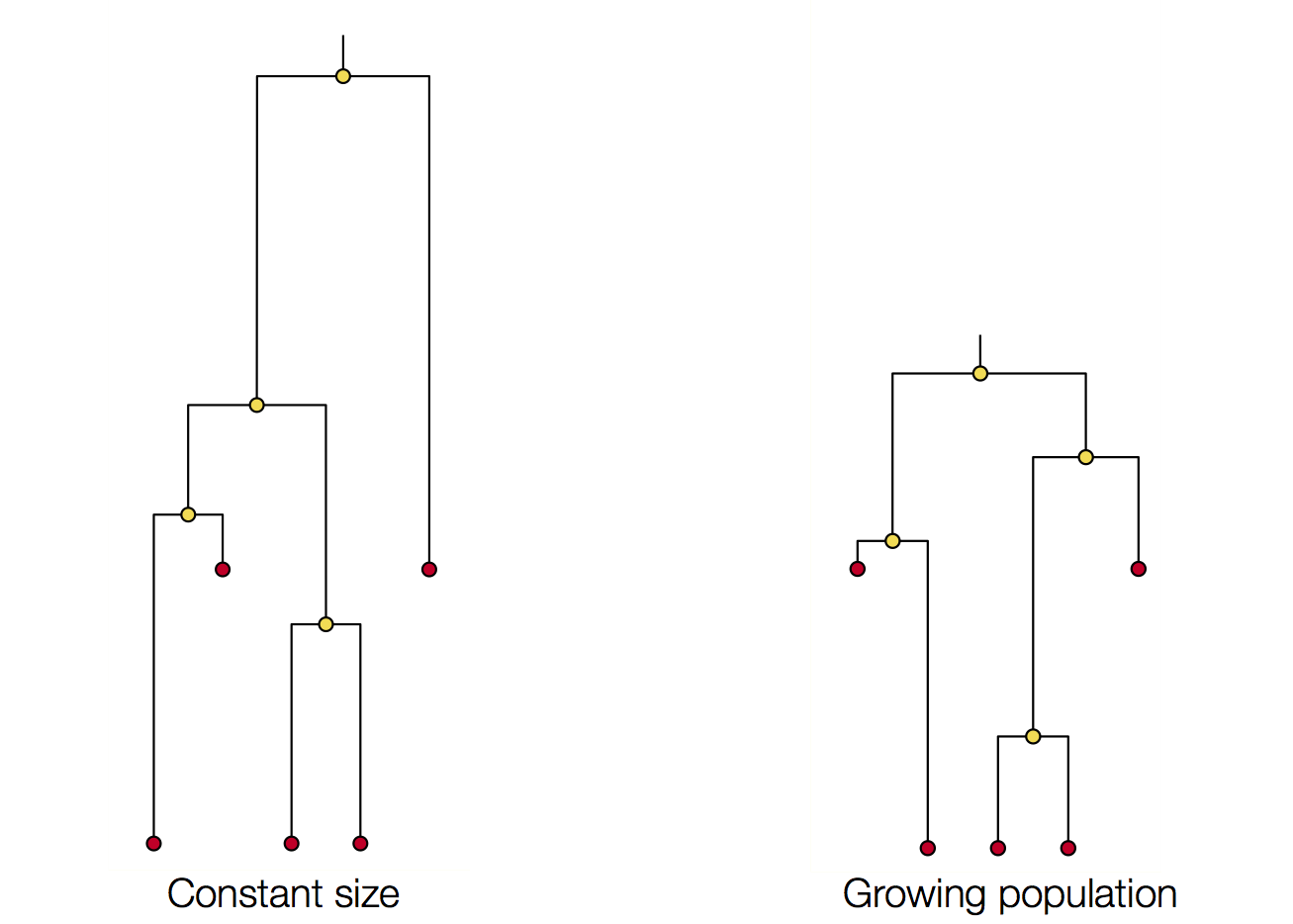### Coalescent simulation exercise.

https://mybinder.org/v2/gh/genomics-course/f12-coalescent/master?filepath=fundamentals-msprime.ipynb

### Coalescent simulation exercise.

https://mybinder.org/v2/gh/genomics-course/f12-coalescent/master?filepath=fundamentals-msprime.ipynb